# Funding Areas

FOR MORE RESEARCH TOPICS

## Problems with the Electroweak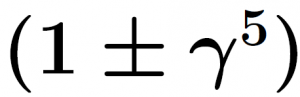Factor

The following discussion relies on these principles:

1. The primary objective of a physical theory is to provide an acceptable prediction and interpretation of experimental results that are carried out within its domain of validity.
2. Quantum mechanics uses a wave-function and operators. An application of an appropriate operator to the wave function that represents a quantum system, yields the required quantity.
3. There is a correspondence between quantum mechanics (QM) and quantum field theory (QFT) (see , p. 49).
4. The Hilbert space is a crucial element of QM (see , pp. 49, 50). The Fock space of QFT is an extension of a Hilbert space of QM .
5. A quantum theory of a specific interaction relies on an appropriate Lagrangian density. The electroweak Lagrangian density contains the factor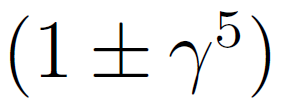which operates on a Dirac spinor (see , p. 308). The following analysis uses the principles 1-5 for an examination of the validity of a more general factor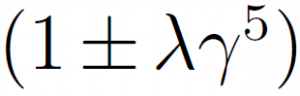, where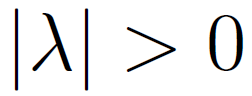is a pure number.

Let us see what happens after applyingto the function of a free motionless spin-up Dirac spinor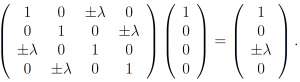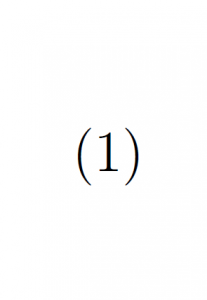Here the notation of the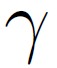matrices is that of , p. 17.

Let us examine the three cases where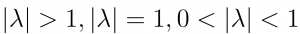.

• If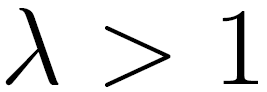then the right-hand side of (1) is a negative-energy Dirac spinor (see , pp. 28-30). It means that in this case the operator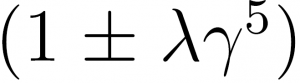projects a massive motionless Dirac particle into an unphysical state. This state is not included in the Hilbert space and expectation values of observables cannot be calculated.
• If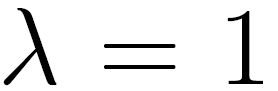then the right-hand side of (1) is a Dirac spinor that has an infinite energy-momentum (see , p. 30). It means that the operator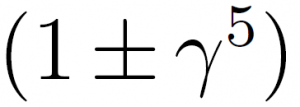projects a massive motionless Dirac particle into an unphysical state and the previous result holds.
•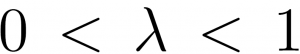then the right-hand side of (1) is a Dirac spinor that moves in the z-direction. The energy of this spinor is greater than that of the original motionless spinor. It follows that in this case the operatorviolates energy conservation.

Conclusion: The electroweak factoris unacceptable.

Research topic: Can the above mentioned discrepancy of the electroweak theory be resolved?

#### References

 S.Weinberg, The Quantum Theory of Fields, Vol. I (Cambridge University Press, Cambridge, 1995).

 https://en.wikipedia.org/wiki/Fock_space

 R. P. Feynman and M. Gell-Mann, Phys. Rev. 109, 193 (1958).

 S. Weinberg, The Quantum Theory of Fields, Vol. II (Cambridge University Press, Cambridge, 1995).

 J. D. Bjorken and S. D. Drell, Relativistic Quantum Mechanics (McGraw-Hill, New York, 1964).